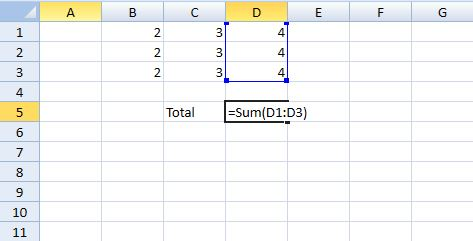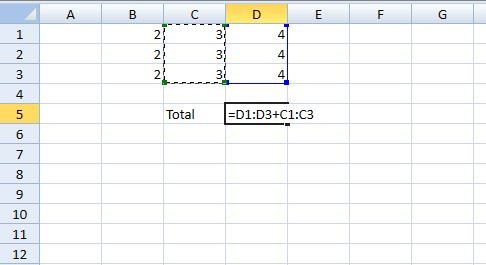﻿ Excel Like formula with Spread for Silverlight# Excel Like formula with Spread for Silverlight

Spread for Silverlight has introduced an exciting new feature. Spread now provides a Formula TextBox which gets enabled when you enter a formula in a cell at run time. It now allows entering formulas in cells just like you do it in Microsoft Excel. Something like the image below:You can drag the selection box to extend the selection to form a Formula range or use functions like Sum(), Product(), etc.To enable the end user to enter formula in a cell you need to set Spread's CanUserEditFormula property to true. End user may start entering a formula in a cell by starting with an equals sign (=). Type the equal sign(=) and then the formula such as = Sum(A1+B1).

``````
You can also put cell reference in a Formula instead of inbuilt functions if you wish to. For example: